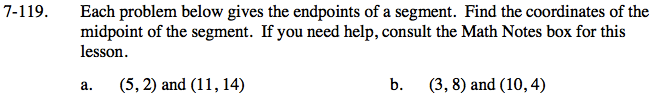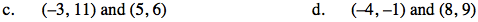### Home > GC > Chapter 7 > Lesson 7.3.3 > Problem7-119

7-119.Average the x-coordinates.

$x = \frac{5 + 11}{2} = 8$

Average the y-coordinates.

$y = \frac{2 + 14}{2} = 8$

The midpoint is at (8,8).

Follow the same procedures to find the midpoints of the other segments.